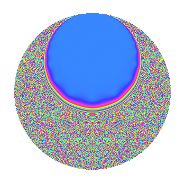Properties

 Label 8.22.a.aLevel 8 Weight 22 Character orbit 8.a Self dual Yes Analytic conductor 22.358 Analytic rank 1 Dimension 2 CM No Inner twists 1

Related objects

Newspace parameters

 Level: $$N$$ = $$8 = 2^{3}$$ Weight: $$k$$ = $$22$$ Character orbit: $$[\chi]$$ = 8.a (trivial)

Newform invariants

 Self dual: Yes Analytic conductor: $$22.358187543$$ Analytic rank: $$1$$ Dimension: $$2$$ Coefficient field: $$\Q(\sqrt{358549})$$ Coefficient ring: $$\Z[a_1, a_2, a_3]$$ Coefficient ring index: $$2^{7}\cdot 3$$ Fricke sign: $$1$$ Sato-Tate group: $\mathrm{SU}(2)$

$q$-expansion

Coefficients of the $$q$$-expansion are expressed in terms of $$\beta = 192\sqrt{358549}$$. We also show the integral $$q$$-expansion of the trace form.

 $$f(q)$$ $$=$$ $$q$$ $$+ ( -52716 - \beta ) q^{3}$$ $$+ ( 1054070 + 20 \beta ) q^{5}$$ $$+ ( 222385896 + 4222 \beta ) q^{7}$$ $$+ ( 5536173789 + 105432 \beta ) q^{9}$$ $$+O(q^{10})$$ $$q$$ $$+ ( -52716 - \beta ) q^{3}$$ $$+ ( 1054070 + 20 \beta ) q^{5}$$ $$+ ( 222385896 + 4222 \beta ) q^{7}$$ $$+ ( 5536173789 + 105432 \beta ) q^{9}$$ $$+ ( 26903201660 + 527181 \beta ) q^{11}$$ $$+ ( -245183338466 - 4314508 \beta ) q^{13}$$ $$+ ( -319917360840 - 2108390 \beta ) q^{15}$$ $$+ ( -3296932336046 - 30718184 \beta ) q^{17}$$ $$+ ( 9651198962660 + 366379451 \beta ) q^{19}$$ $$+ ( -67527792412128 - 444952848 \beta ) q^{21}$$ $$+ ( -204868932888136 - 944258822 \beta ) q^{23}$$ $$+ ( -470439074503825 + 42162800 \beta ) q^{25}$$ $$+ ( -1133969725036728 - 633773898 \beta ) q^{27}$$ $$+ ( -1202393761072530 + 23192603812 \beta ) q^{29}$$ $$+ ( -4344953585279584 - 18061983176 \beta ) q^{31}$$ $$+ ( -8386270582391376 - 54694075256 \beta ) q^{33}$$ $$+ ( 1350500251768560 + 8898001460 \beta ) q^{35}$$ $$+ ( -1093102048125930 - 50855746684 \beta ) q^{37}$$ $$+ ( 69952311535648344 + 472626942194 \beta ) q^{39}$$ $$+ ( 34089019286779338 - 13526799376 \beta ) q^{41}$$ $$+ ( 132264826133002012 - 769879839131 \beta ) q^{43}$$ $$+ ( 33706570046274270 + 221856184020 \beta ) q^{45}$$ $$+ ( 213247205779311216 - 2319694970380 \beta ) q^{47}$$ $$+ ( -273483788820065767 + 1877826505824 \beta ) q^{49}$$ $$+ ( 579820228277510760 + 4916272123790 \beta ) q^{51}$$ $$+ ( -1527990137794759066 + 2918184305860 \beta ) q^{53}$$ $$+ ( 167718685847412520 + 1093749709870 \beta ) q^{55}$$ $$+ ( -5351411440184130096 - 28965258101576 \beta ) q^{57}$$ $$+ ( 391712498761407212 - 7304311733439 \beta ) q^{59}$$ $$+ ( -3588639524539298546 + 23337258872596 \beta ) q^{61}$$ $$+ ( 7114746750858671688 + 46820315524230 \beta ) q^{63}$$ $$+ ( -1398984934878350380 - 9451460216880 \beta ) q^{65}$$ $$+ ( 8337061587005769044 - 77794800439793 \beta ) q^{67}$$ $$+ ( 23280659176128041568 + 254646480948688 \beta ) q^{69}$$ $$+ ( 4724131574924358184 - 231241233933906 \beta ) q^{71}$$ $$+ ( -5793070167251503766 - 528252850485192 \beta ) q^{73}$$ $$+ ( 24242377320236937900 + 468216420339025 \beta ) q^{75}$$ $$+ ( 35401963412776636512 + 230822936447696 \beta ) q^{77}$$ $$+ ( -42640351109357948912 + 355065026452268 \beta ) q^{79}$$ $$+ ( 10244953197363286809 + 64523790945000 \beta ) q^{81}$$ $$+ ( -190907311020043122908 - 591846679323509 \beta ) q^{83}$$ $$+ ( -11595580332466203700 - 98317762929800 \beta ) q^{85}$$ $$+ ( -243164018799315989352 - 20227541480862 \beta ) q^{87}$$ $$+ ( -29871466215347989830 - 1314255056990664 \beta ) q^{89}$$ $$+ ( -295294267388978008272 - 1994649782382620 \beta ) q^{91}$$ $$+ ( 467783744998363697280 + 5297109090385600 \beta ) q^{93}$$ $$+ ( 107025816003941936920 + 579213567168770 \beta ) q^{95}$$ $$+ ( 391697330463355975394 + 156001529627000 \beta ) q^{97}$$ $$+ ( 883595341143359946252 + 5755023991675929 \beta ) q^{99}$$ $$+O(q^{100})$$ $$\operatorname{Tr}(f)(q)$$ $$=$$ $$2q$$ $$\mathstrut -\mathstrut 105432q^{3}$$ $$\mathstrut +\mathstrut 2108140q^{5}$$ $$\mathstrut +\mathstrut 444771792q^{7}$$ $$\mathstrut +\mathstrut 11072347578q^{9}$$ $$\mathstrut +\mathstrut O(q^{10})$$ $$2q$$ $$\mathstrut -\mathstrut 105432q^{3}$$ $$\mathstrut +\mathstrut 2108140q^{5}$$ $$\mathstrut +\mathstrut 444771792q^{7}$$ $$\mathstrut +\mathstrut 11072347578q^{9}$$ $$\mathstrut +\mathstrut 53806403320q^{11}$$ $$\mathstrut -\mathstrut 490366676932q^{13}$$ $$\mathstrut -\mathstrut 639834721680q^{15}$$ $$\mathstrut -\mathstrut 6593864672092q^{17}$$ $$\mathstrut +\mathstrut 19302397925320q^{19}$$ $$\mathstrut -\mathstrut 135055584824256q^{21}$$ $$\mathstrut -\mathstrut 409737865776272q^{23}$$ $$\mathstrut -\mathstrut 940878149007650q^{25}$$ $$\mathstrut -\mathstrut 2267939450073456q^{27}$$ $$\mathstrut -\mathstrut 2404787522145060q^{29}$$ $$\mathstrut -\mathstrut 8689907170559168q^{31}$$ $$\mathstrut -\mathstrut 16772541164782752q^{33}$$ $$\mathstrut +\mathstrut 2701000503537120q^{35}$$ $$\mathstrut -\mathstrut 2186204096251860q^{37}$$ $$\mathstrut +\mathstrut 139904623071296688q^{39}$$ $$\mathstrut +\mathstrut 68178038573558676q^{41}$$ $$\mathstrut +\mathstrut 264529652266004024q^{43}$$ $$\mathstrut +\mathstrut 67413140092548540q^{45}$$ $$\mathstrut +\mathstrut 426494411558622432q^{47}$$ $$\mathstrut -\mathstrut 546967577640131534q^{49}$$ $$\mathstrut +\mathstrut 1159640456555021520q^{51}$$ $$\mathstrut -\mathstrut 3055980275589518132q^{53}$$ $$\mathstrut +\mathstrut 335437371694825040q^{55}$$ $$\mathstrut -\mathstrut 10702822880368260192q^{57}$$ $$\mathstrut +\mathstrut 783424997522814424q^{59}$$ $$\mathstrut -\mathstrut 7177279049078597092q^{61}$$ $$\mathstrut +\mathstrut 14229493501717343376q^{63}$$ $$\mathstrut -\mathstrut 2797969869756700760q^{65}$$ $$\mathstrut +\mathstrut 16674123174011538088q^{67}$$ $$\mathstrut +\mathstrut 46561318352256083136q^{69}$$ $$\mathstrut +\mathstrut 9448263149848716368q^{71}$$ $$\mathstrut -\mathstrut 11586140334503007532q^{73}$$ $$\mathstrut +\mathstrut 48484754640473875800q^{75}$$ $$\mathstrut +\mathstrut 70803926825553273024q^{77}$$ $$\mathstrut -\mathstrut 85280702218715897824q^{79}$$ $$\mathstrut +\mathstrut 20489906394726573618q^{81}$$ $$\mathstrut -\mathstrut 381814622040086245816q^{83}$$ $$\mathstrut -\mathstrut 23191160664932407400q^{85}$$ $$\mathstrut -\mathstrut 486328037598631978704q^{87}$$ $$\mathstrut -\mathstrut 59742932430695979660q^{89}$$ $$\mathstrut -\mathstrut 590588534777956016544q^{91}$$ $$\mathstrut +\mathstrut 935567489996727394560q^{93}$$ $$\mathstrut +\mathstrut 214051632007883873840q^{95}$$ $$\mathstrut +\mathstrut 783394660926711950788q^{97}$$ $$\mathstrut +\mathstrut 1767190682286719892504q^{99}$$ $$\mathstrut +\mathstrut O(q^{100})$$

Embeddings

For each embedding $$\iota_m$$ of the coefficient field, the values $$\iota_m(a_n)$$ are shown below.

For more information on an embedded modular form you can click on its label.

Label $$\iota_m(\nu)$$ $$a_{2}$$ $$a_{3}$$ $$a_{4}$$ $$a_{5}$$ $$a_{6}$$ $$a_{7}$$ $$a_{8}$$ $$a_{9}$$ $$a_{10}$$
1.1
 299.895 −298.895
0 −167684. 0 3.35342e6 0 7.07779e8 0 1.76574e10 0
1.2 0 62251.6 0 −1.24528e6 0 −2.63007e8 0 −6.58509e9 0
 $$n$$: e.g. 2-40 or 990-1000 Significant digits: Format: Complex embeddings Normalized embeddings Satake parameters Satake angles

Inner twists

This newform does not admit any (nontrivial) inner twists.

Atkin-Lehner signs

$$p$$ Sign
$$2$$ $$1$$

Hecke kernels

This newform can be constructed as the kernel of the linear operator $$T_{3}^{2}$$ $$\mathstrut +\mathstrut 105432 T_{3}$$ $$\mathstrut -\mathstrut 10438573680$$ acting on $$S_{22}^{\mathrm{new}}(\Gamma_0(8))$$.